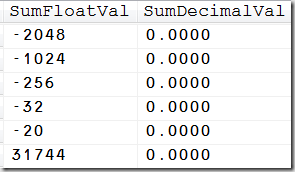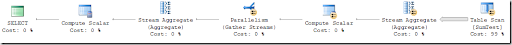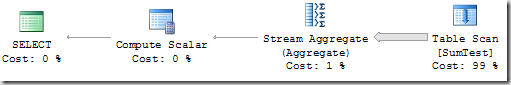# Did you know: Aggregate functions on floats may be non-deterministic

One day some of the report-users mentioned that, every time they run a report, they get different results. My first idea was that there were some undergoing data changes, probably from a different connection/user, so this would explain it. But it turned out that no modifications were made. Even setting the database to read only did not help. Numbers in reports differed by about 20% with every execution.

Delving into it, I could isolate the problem. It was a single SELECT statement that, when invoked, returned different results. The numbers differed by up to 20% in value without any data changes being performed!

Have a look at the following sample. We create a test table to demonstrate what I'm talking about:

 use tempdb go if (object_id('SumTest', 'U') is not null) drop table SumTest go create table SumTest ( floatVal float not null ,decimalVal decimal(20,4) not null ,filler nchar(300) not null default '#' ) go

The table has three columns, where the third column only serves the purpose of filling up the row, so the table contains more data pages.

Let's now insert 600000 rows into our table:

 declare @x float set @x = 1000000000000000.9999 -- Insert 300000 identical rows insert SumTest(floatVal, decimalVal) select top(300000) @x, @x from sys.trace_event_bindings as b1 ,sys.trace_event_bindings as b2 -- Again insert 300000 rows. -- This time with negative sign insert SumTest(floatVal, decimalVal) select top(300000) -@x, -@x from sys.trace_event_bindings as b1 ,sys.trace_event_bindings as b2

The first INSERT statement adds 300000 rows with positive values for the two columns floatVal and decimalVal. After that, we insert another 300000 rows, this time with inverse signs. So in total, values for each of the two columns should add up to zero. Let's check this by invoking the summation over all rows a few times:

 select sum(floatVal) as SumFloatVal ,sum(decimalVal) as SumDecimalVal from SumTest union select sum(floatVal) as SumFloatVal ,sum(decimalVal) as SumDecimalVal from SumTest union select sum(floatVal) as SumFloatVal ,sum(decimalVal) as SumDecimalVal from SumTest union select sum(floatVal) as SumFloatVal ,sum(decimalVal) as SumDecimalVal from SumTest union select sum(floatVal) as SumFloatVal ,sum(decimalVal) as SumDecimalVal from SumTest union select sum(floatVal) as SumFloatVal ,sum(decimalVal) as SumDecimalVal from SumTest union select sum(floatVal) as SumFloatVal ,sum(decimalVal) as SumDecimalVal from SumTest union select sum(floatVal) as SumFloatVal ,sum(decimalVal) as SumDecimalVal from SumTest union select sum(floatVal) as SumFloatVal ,sum(decimalVal) as SumDecimalVal from SumTest

And here's the result:As for the DECIMAL column, the outcome is as expected. But look at the totals for the FLOAT column. It's perfectly understandable, the sum will reveal some rounding errors. What really puzzled me is the difference between the numbers. Why isn't the rounding error the same for all executions?

I was pretty sure that I discovered a bug in SQL Server and posted a regarding item on MSFT's connect platform (see here).

Unfortunately nobody cared about my problem, and so I took the opportunity of talking to some fellows of the SQL Server CAT team on the occasion of the 2009 PASS Summit. After a while, I received an explanation which I'd like to repeat here.

The query is executed in parallel, as the plan reveals:When summing up values, usually the summation sequence doesn't matter. (If you remember some mathematics from school that's what the commutative law of addition is about). Therefore, reading values in multiple threads and adding up the values in any arbitrary order is perfect, as the order doesn't have any influence on the result. Well, at least theoretically. When adding float values, there's floating point arithmetic rounding errors with every addition. These added-up rounding errors are the reason for the non-zero values of the float totals in our example. So that's ok, but why different results with almost every execution? The reason for this is parallel execution. Added-up rounding errors depend on the sequence, so the commutative law does not really apply to these errors. There's a chance that the sequence of rows changes with every execution, if the query is executed in parallel. And that's why the results change, dependent only on some butterfly wing movements at the other side of the world.

If we add the MAXDOP 1 query hint, only one thread is utilized and the results are the same for every execution, although rounding errors still remain present. So this query:

 select sum(floatVal) as SumFloatVal ,sum(decimalVal) as SumDecimalVal from SumTest option (maxdop 1)

will be executed by using the following (single thread) execution plan:This time the result (and also the rounding error) is always the same.

Pretty soon after delivering the explanation, the bug was closed. Reason: the observed behavior is "by design".

I can understand that the problem originates from computer resp. processor architecture and MSFT has no chance of control therefore.

Although.

When using SSAS' write back functionality, SSAS will always create numeric columns of FLOAT data types. There's no chance of manipulating the data type; it's always float!

Additionally, SSAS more often than not inserts rows into write back tables with vastly large resp. small values. When looking an these rows, it appeared that they are created solely with the intention of summing up to zero. We discovered plenty of these rows containing inverse values that usually should nullify in total, but apparently don't. By the way that's why closing the bug with the "By Design" explanation makes me somewhat sad.

So, probably avoiding FLOATs is a good idea! Unfortunately, this is simply not possible in all cases and sometimes out of our control.